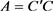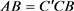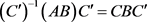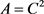﻿ 对称正定矩阵的分解

# 对称正定矩阵的分解Decomposition of Symmetric Positive Definite Matrices

Abstract: Based on the special properties of symmetric positive definite matrix, the decomposition of symmetric positive definite matrix is extended by using three different ideas: spectral decomposi-tion, Lagrange interpolation and commutativity of matrix, and it is applied to solve more mathe-matical problems.

1. 引言

$A\in {R}^{n×n}$ ，若

$A={A}^{\text{T}}$

$0\ne X\in {R}^{n}$

${X}^{\text{T}}AX>0$(1)

1) 若A正定或B正定，则AB的特征值全是实数；

2) 若A正定，则B正定的充要条件是AB的特征值全是正实数；

3) 若A，B都半正定，则AB的特征值都是非负实数。

$A={C}^{\prime }C$.. (2). (3)

(2)与(3)用于与(1)类似的思想解决，唯一不同的是(3)中的半正定矩阵矩阵只能分解为两个实矩阵的乘积，但不能确保是可逆矩阵，但是对任意正实数t， $A+t{E}_{n}$ 是正定矩阵，所以利用多项式对t的连续性，则可以较容易得到结论。∎

2. 命题的证明与推广

${T}^{\prime }AT=\left(\begin{array}{cccc}{\lambda }_{1}& & & \\ & {\lambda }_{2}& & \\ & & \ddots & \\ & & & {\lambda }_{n}\end{array}\right)$

${\lambda }_{i}>0,i=1,\cdots ,n$.

$A=T\left(\begin{array}{cccc}{\lambda }_{1}& & & \\ & {\lambda }_{2}& & \\ & & \ddots & \\ & & & {\lambda }_{n}\end{array}\right){T}^{\prime }=T\left(\begin{array}{cccc}\sqrt{{\lambda }_{1}}& & & \\ & \sqrt{{\lambda }_{2}}& & \\ & & \ddots & \\ & & & \sqrt{{\lambda }_{n}}\end{array}\right){T}^{\prime }T\left(\begin{array}{cccc}\sqrt{{\lambda }_{1}}& & & \\ & \sqrt{{\lambda }_{2}}& & \\ & & \ddots & \\ & & & \sqrt{{\lambda }_{n}}\end{array}\right){T}^{\prime }$ ,

$C=T\left(\begin{array}{cccc}\sqrt{{\lambda }_{1}}& & & \\ & \sqrt{{\lambda }_{2}}& & \\ & & \ddots & \\ & & & \sqrt{{\lambda }_{n}}\end{array}\right){T}^{\prime }$，且C是一个对称正定矩阵。

${\lambda }_{1},{\lambda }_{2},\cdots ,{\lambda }_{k}$$\phi$ 的全体不同的特征值， ${W}_{i}$$\phi$ 属于 ${\lambda }_{i}$ 的特征子空间，则V是 ${W}_{i}\left(i=1,\cdots ,k\right)$ 的正交直和。

${E}_{i}$ 是V到 ${W}_{i}$ 上的正交投影，则 $\phi$ 有下列分解式：

$\phi ={\lambda }_{1}{E}_{1}+{\lambda }_{2}{E}_{2}+\cdots +{\lambda }_{m}{E}_{m}$

${\phi }^{k}={\lambda }_{1}^{k}{E}_{1}+{\lambda }_{2}^{k}{E}_{2}+\cdots +{\lambda }_{m}^{k}{E}_{m}$

$\phi ={\lambda }_{1}{E}_{1}+{\lambda }_{2}{E}_{2}+\cdots +{\lambda }_{m}{E}_{m}$

${d}_{i}={\lambda }_{i}^{1/k}\left(i=1,2,3,\cdots ,m\right)$

$\psi ={d}_{1}{E}_{1}+{d}_{2}{E}_{2}+\cdots +{d}_{m}{E}_{m}$

${\theta }^{k}=\phi$

$\theta ={b}_{1}{F}_{1}+{b}_{2}{F}_{2}+\cdots +{b}_{r}{F}_{r}$

$\theta$ 的谱分解式，其中 ${F}_{i}$ 为正交投影算子，且 ${b}_{i}$ 为正实数。

${\theta }^{k}=\phi$$\phi ={\sum }_{i=1}^{r}{b}_{i}^{K}{F}_{i}$

$r=m$${b}_{i}={d}_{i}$

${F}_{i}={E}_{i}$$\psi =\theta$

${\lambda }_{i}^{k}\left(i=1,\cdots ,m\right)$

Step 1

$A=P\left(\begin{array}{cccc}{\lambda }_{1}& & & \\ & \ddots & & \\ & & \ddots & \\ & & & {\lambda }_{n}\end{array}\right){P}^{\prime }$(*)

$P\left(\begin{array}{cccc}{\lambda }_{1}^{\frac{1}{k}}& & & \\ & \ddots & & \\ & & \ddots & \\ & & & {\lambda }_{n}^{\frac{1}{k}}\end{array}\right){P}^{\prime }=f\left(A\right)$

$f\left(\lambda \right)$$s-1$ 次多项式，且 ${\lambda }_{i}^{\frac{1}{k}}=f\left({\lambda }_{i}\right)$ ；( $i=1,\cdots ,s$ )，故得：

$\left(\begin{array}{cccc}{\lambda }_{1}^{\frac{1}{k}}& & & \\ & \ddots & & \\ & & \ddots & \\ & & & {\lambda }_{n}^{\frac{1}{k}}\end{array}\right)=\left(\begin{array}{cccc}f\left({\lambda }_{1}\right)& & & \\ & \ddots & & \\ & & \ddots & \\ & & & f\left({\lambda }_{n}\right)\end{array}\right)=f\left(\left(\begin{array}{cccc}{\lambda }_{1}& & & \\ & \ddots & & \\ & & \ddots & \\ & & & {\lambda }_{n}\end{array}\right)\right)$

$P\left(\begin{array}{cccc}{\lambda }_{1}^{\frac{1}{k}}& & & \\ & \ddots & & \\ & & \ddots & \\ & & & {\lambda }_{n}^{\frac{1}{k}}\end{array}\right){P}^{\prime }=Pf\left(\left(\begin{array}{cccc}{\lambda }_{1}& & & \\ & \ddots & & \\ & & \ddots & \\ & & & {\lambda }_{n}\end{array}\right)\right){P}^{\prime }=f\left(P\left(\begin{array}{cccc}{\lambda }_{1}& & & \\ & \ddots & & \\ & & \ddots & \\ & & & {\lambda }_{n}\end{array}\right){P}^{\prime }\right)=f\left(A\right)$

Step 2

$B=P\left(\begin{array}{cccc}{\lambda }_{1}^{\frac{1}{k}}& & & \\ & \ddots & & \\ & & \ddots & \\ & & & {\lambda }_{n}^{\frac{1}{k}}\end{array}\right){P}^{\prime }$${{P}^{\prime }}_{1}B{P}_{1}=\left(\begin{array}{cccc}{\lambda }_{1}^{\frac{1}{k}}{E}_{{r}_{1}}& & & \\ & \ddots & & \\ & & \ddots & \\ & & & {\lambda }_{t}^{\frac{1}{k}}{E}_{{r}_{t}}\end{array}\right)$ , ${{P}^{\prime }}_{2}C{P}_{2}=\left(\begin{array}{cccc}{\lambda }_{1}^{\frac{1}{k}}{E}_{{r}_{1}}& & & \\ & \ddots & & \\ & & \ddots & \\ & & & {\lambda }_{t}^{\frac{1}{k}}{E}_{{r}_{t}}\end{array}\right)$

${B}^{k}={C}^{k}$ ，且

${B}^{k}={P}_{1}\left(\begin{array}{cccc}{\lambda }_{1}{E}_{{r}_{1}}& & & \\ & \ddots & & \\ & & \ddots & \\ & & & {\lambda }_{t}{E}_{{r}_{t}}\end{array}\right){{P}^{\prime }}_{1}$ , ${C}^{k}={P}_{2}\left(\begin{array}{cccc}{\lambda }_{1}{E}_{{r}_{1}}& & & \\ & \ddots & & \\ & & \ddots & \\ & & & {\lambda }_{t}{E}_{{r}_{t}}\end{array}\right){{P}^{\prime }}_{2}$

${{P}^{\prime }}_{2}{P}_{1}\left(\begin{array}{cccc}{\lambda }_{1}{E}_{{r}_{1}}& & & \\ & \ddots & & \\ & & \ddots & \\ & & & {\lambda }_{t}{E}_{{r}_{t}}\end{array}\right)=\left(\begin{array}{cccc}{\lambda }_{1}{E}_{{r}_{1}}& & & \\ & \ddots & & \\ & & \ddots & \\ & & & {\lambda }_{t}{E}_{{r}_{t}}\end{array}\right){{P}^{\prime }}_{2}{P}_{1}$

$\left(\begin{array}{cccc}{\lambda }_{1}{E}_{{r}_{1}}& & & \\ & \ddots & & \\ & & \ddots & \\ & & & {\lambda }_{t}{E}_{{r}_{t}}\end{array}\right)$

${{P}^{\prime }}_{2}{P}_{1}=\left(\begin{array}{cccc}{B}_{1}& & & \\ & \ddots & & \\ & & \ddots & \\ & & & {B}_{t}\end{array}\right)$

${B}_{i}$ 为分块对角矩阵， $i=1,2,\cdots ,t$ ，则 ${{P}^{\prime }}_{2}{P}_{1}$ 与分块对角矩阵 $\left(\begin{array}{cccc}{\lambda }_{1}^{\frac{1}{k}}{E}_{{r}_{1}}& & & \\ & \ddots & & \\ & & \ddots & \\ & & & {\lambda }_{t}^{\frac{1}{k}}{E}_{{r}_{t}}\end{array}\right)$ 也可交换。

${{P}^{\prime }}_{2}{P}_{1}\left(\begin{array}{cccc}{\lambda }_{1}^{\frac{1}{k}}{E}_{{r}_{1}}& & & \\ & \ddots & & \\ & & \ddots & \\ & & & {\lambda }_{t}^{\frac{1}{k}}{E}_{{r}_{t}}\end{array}\right)=\left(\begin{array}{cccc}{\lambda }_{1}^{\frac{1}{k}}{E}_{{r}_{1}}& & & \\ & \ddots & & \\ & & \ddots & \\ & & & {\lambda }_{t}^{\frac{1}{k}}{E}_{{r}_{t}}\end{array}\right){{P}^{\prime }}_{2}{P}_{1}$

${P}_{1}\left(\begin{array}{cccc}{\lambda }_{1}^{\frac{1}{k}}{E}_{{r}_{1}}& & & \\ & \ddots & & \\ & & \ddots & \\ & & & {\lambda }_{t}^{\frac{1}{k}}{E}_{{r}_{t}}\end{array}\right){{P}^{\prime }}_{1}={P}_{2}\left(\begin{array}{cccc}{\lambda }_{1}^{\frac{1}{k}}{E}_{{r}_{1}}& & & \\ & \ddots & & \\ & & \ddots & \\ & & & {\lambda }_{t}^{\frac{1}{k}}{E}_{{r}_{t}}\end{array}\right){{P}^{\prime }}_{2}$

$B=C$。∎

3. 命题的应用

$Ax=b$

${a}_{ii}\ne 0,i=1,2,\cdots ,n$.

$A=D-L-U$

$D=\left(\begin{array}{ccccc}{a}_{11}& & & & \\ & {a}_{22}& & & \\ & & \ddots & & \\ & & & {a}_{n-1n-1}& \\ & & & & {a}_{nn}\end{array}\right)$

$L=\left(\begin{array}{ccccc}0& & & & \\ -{a}_{21}& 0& & & \\ -{a}_{31}& -{a}_{32}& \ddots & & \\ ⋮& ⋮& ⋮& 0& \\ -{a}_{n1}& -{a}_{n2}& \cdots & -{a}_{n,n-1}& 0\end{array}\right)$

$U=\left(\begin{array}{ccccc}0& -{a}_{12}& -{a}_{13}& \cdots & -{a}_{21}\\ & 0& -{a}_{23}& \cdots & -{a}_{21}\\ & & 0& \cdots & ⋮\\ & & & \ddots & -{a}_{n-1,n}\\ & & & & 0\end{array}\right)$

$DX=\left(L+U\right)X+b$

$J={D}^{-1}\left(L+U\right)$ , $f={D}^{-1}b$

${x}^{\left(k+1\right)}=J{x}^{\left(k\right)}+f,k=0,1,\cdots$

$D=diag\left({a}_{11},{a}_{22},\cdots ,{a}_{nn}\right)$

$AX=b$ (其中 $A\in {M}^{n×n}$$b\in I{R}^{n}$ )${a}_{ii}>0$

${D}^{-1/2}x\ne 0$

${\left({D}^{-1/2}x\right)}^{\text{T}}A\left({D}^{-1/2}x\right)>0,\text{\hspace{0.17em}}{x}^{\text{T}}{\left({D}^{-1/2}\right)}^{\text{T}}A{D}^{-1/2}x>0$

${D}^{-1/2}A{D}^{-1/2}$ 为正定阵，所以 ${E}_{n}-{D}^{-1/2}A{D}^{-1/2}$ 特征值均小于1。

${E}_{n}-{D}^{-1/2}\left(2D-A\right){D}^{-1/2}=-\left({E}_{n}-{D}^{-1/2}A{D}^{-1/2}\right)$

$|\rho \left(J\right)|<1$

$B=P-{H}^{\text{T}}PH$

${x}^{\left(k+1\right)}=H{x}^{\left(k\right)}+b,k=0,1,\cdots$.

$P={L}^{1/2}{L}^{1/2}$ ,

$\begin{array}{c}{L}^{-1/2}B{L}^{-1/2}={L}^{-1/2}\left(P-{H}^{\text{T}}PH\right){L}^{-1/2}\\ ={E}_{n}-{L}^{-1/2}{H}^{\text{T}}PH{L}^{-1/2}\\ ={E}_{n}-{L}^{-1/2}{H}^{\text{T}}{L}^{1/2}{L}^{1/2}H{L}^{-1/2}\end{array}$

$C={L}^{-1/2}{H}^{\text{T}}{L}^{1/2}$

${C}^{\text{T}}={\left({L}^{1/2}\right)}^{\text{T}}H{\left({L}^{-1/2}\right)}^{\text{T}}$

${C}^{\text{T}}=C$

${E}_{n}-C{C}^{\text{T}}$ 的特征值均大于0，即C特征值的平方小于1，迭代格式收敛。∎

${\left(P{P}^{\prime }\right)}^{\frac{1}{2}}A=A{\left(P{P}^{\prime }\right)}^{\frac{1}{2}}$.

${{P}^{\prime }}_{1}B{P}_{1}=A$.

${\phi }^{*}=g\left(\phi \right)$

$f\left(A\right)=f\left({{P}^{\prime }}_{1}B{P}_{1}\right)={{P}^{\prime }}_{1}f\left(B\right){P}_{1}={{P}^{\prime }}_{1}{B}^{\prime }{P}_{1}={\left({P}_{1}B{{P}^{\prime }}_{1}\right)}^{\prime }={A}^{\prime }$

${A}^{\prime }=f\left(A\right)$.

$\left(P{{P}^{\prime }}^{\frac{1}{2}}\right)=g\left(P{P}^{\prime }\right)$

$g\left(P{P}^{\prime }\right)A=Ag\left(P{P}^{\prime }\right)$

$P{P}^{\prime }A=AP{P}^{\prime }$

${\left(P{P}^{\prime }\right)}^{\frac{1}{2}}A=A{\left(P{P}^{\prime }\right)}^{\frac{1}{2}}$

$P{P}^{\prime }A=AP{P}^{\prime }$

$P{P}^{\prime }{A}^{\prime }={A}^{\prime }P{P}^{\prime }$

$P{P}^{\prime }f\left(A\right)=f\left(A\right)P{P}^{\prime }$

${P}^{-1}f\left(A\right)P=f\left({P}^{-1}AP\right)=f\left(B\right)={B}^{\prime }={P}^{\prime }{A}^{\prime }{\left({P}^{-1}\right)}^{\prime }={P}^{\prime }f\left(A\right){\left({P}^{-1}\right)}^{\prime }$

${P}^{-1}f\left(A\right)P={P}^{\prime }f\left(A\right){\left({P}^{-1}\right)}^{\prime }$

$P{P}^{\prime }f\left(A\right)=f\left(A\right)P{P}^{\prime }$

4. 结语

NOTES

*并列第一作者。

 姚慕生, 谢启鸿. 高等代数[M]. 第三版. 上海: 复旦大学出版社, 2015: 458-459.

 姚慕生, 吴泉水, 谢启鸿. 高等代数学[M]. 第三版. 上海: 复旦大学出版社, 2014: 408-410.

 屠伯埙. 线性代数——方法导引[M]. 上海: 复旦大学出版社, 1986: 184-186.

 孙文瑜, 杜其奎. 计算方法[M]. 北京: 科学出版社, 2007: 53-74.

 屠伯埙. 线性代数——方法导引[M]. 上海: 复旦大学出版社, 1986: 194.

 姚慕生, 谢启鸿. 高等代数[M]. 第三版. 上海: 复旦大学出版社, 2015: 480.

Top